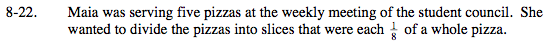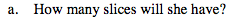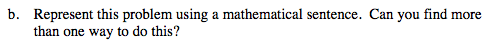### Home > MC2 > Chapter 8 > Lesson 8.1.2 > Problem8-22

8-22.$\text{How many } \frac{1}{8} \text{ slices of pizza equal a whole?}$

Let x represent the number of slices in a whole pizza.

$\frac{1}{8} (x) = 1$

There are 8 slices in each whole pizza.
If there are 5 whole pizzas, how many slices are there total?

40 slices.$5 \div \frac{1}{8} = 40$

Now try to find another way to arrive at this answer.